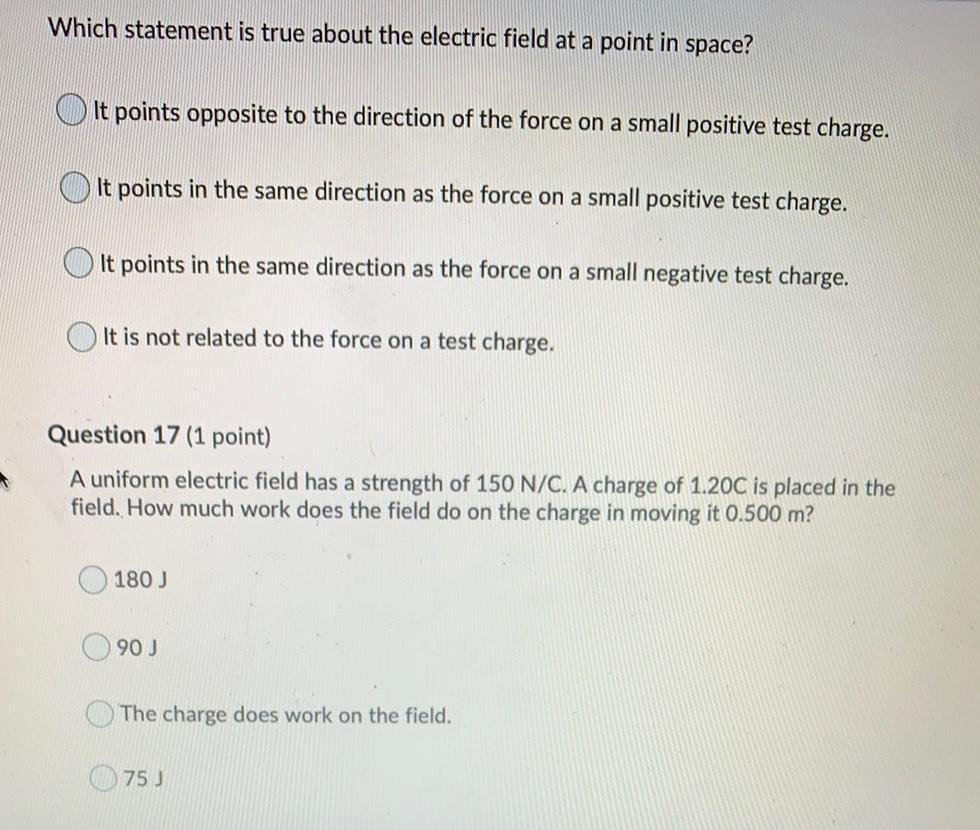Question:

# Which statement is true about the electric field at a point in space? It points opposite to the direction of the force on a smalWhich statement is true about the electric field at a point in space? It points opposite to the direction of the force on a small positive test charge. It points in the same direction as the force on a small positive test charge. It points in the same direction as the force on a small negative test charge. It is not related to the force on a test charge. Question 17 (1 point) A uniform electric field has a strength of 150 N/C. A charge of 1.20C is placed in the field. How much work does the field do on the charge in moving it 0.500 m? 180) 90 J The charge does work on the field. 75